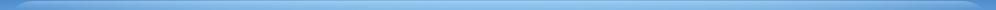学术活动学术会议前沿科学论坛Colloquium专题学术报告/SeminarLunch SeminarCoffee Time研究生高级课程其他学术活动您现在的位置：首页 > 学术活动 > 专题学术报告/Seminar
 (Seminar) Stringy Effects at Low-Energy Limit
 2019-09-03  【大 中 小】【打印】【关闭】

CAS Key Laboratory of Theoretical Physics

Institute of Theoretical Physics

Seminar

Title

 Stringy Effects at Low-Energy Limit

Speaker

 Chen-Te Ma (马承德)

Affiliation

South China Normal University & University of Cape Town

Date

September 3 (Tuesday), 2019, 14:30-16:00

Venue

ITP South Building 6420

Abstract

In the simple case of bosonic closed string theory with one of the twenty-five spatial directions being a circle of radius $R$, one has to take the field-theory limit $R\gg\sqrt{\alpha^{\prime}}$ to study the low-energy physics. The corresponding massless spectrum, which does not have the winding number and internal momenta excitation, is obtained when the left-moving and right-moving oscillator numbers equal to one, i.e.  $N_L=N_R=1$. This condition characterizes the strong constraint solutions in Double Field Theory. When, in the context of this letter,  one considers instead $N_L\neq N_R$, then the strong constraint solution is not enough to take into account the massless states with nontrivial internal momentum and winding number. This stringy effect in the low-energy limit should be expected from a perturbation in the parameter $\sqrt{\alpha^{\prime}}/R$. We use a linear gauge transformation to construct the quadratic action with a new mass term for the state $(N_L, N_R)=(2, 0), (0, 2)$, and the action is T-dual invariant. By obtaining a gauge transformation for a non-linear extension, a constraint acting on fields can be uniquely defined in triple-products. Finally, we show that the stringy effects only appear in the higher dimensional compact dimensions.

Contact Person

 冼卓宇IE6.0浏览器，1024X768分辨率 版权所有 ？ 中国科学院理论物理研究所 地址：北京市海淀区中关村东路55号　邮政编码：100190【京ICP备05002865号】 京公网安备1101080094号